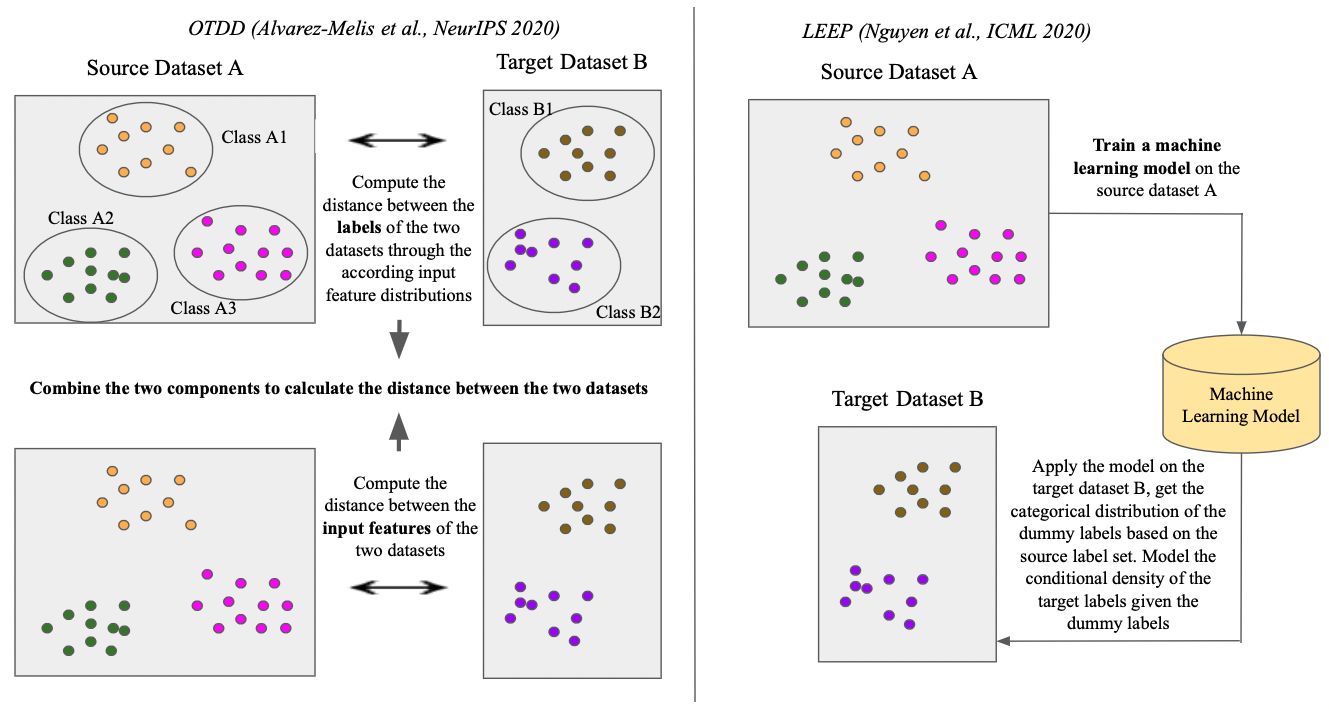## Overview

• Transfer learning has become a popular approach when it comes to utilizing pre-trained models for new downstream tasks. However, efficiently evaluating the transferability among different datasets is still a popular research topic.
• Suppose there are two datasets: the source dataset and the target dataset, and we’re interested in evaluating the transferability of machine learning models from the source dataset to the target dataset.
• Transferability estimation seeks to help select a pre-trained model for transfer learning, so as to maximize performance on the target task and prevent negative transfer.
• Let’s delve deeper into two proposals that perform transferability estimation: Comparative Summary between Log Expected Empirical Prediction (LEEP) and Optimal Transport Dataset Distance (OTDD)
• The following article has been written by Zhibo Zhang with contributions from Aman Chadha.

### Log Expected Empirical Prediction (LEEP)

• LEEP by Nguyen et al. from Amazon Web Services and Facebook AI in ICML 2020 proposes to measure the transferability from the source dataset to the target dataset by evaluating the log likelihood of the correct prediction on the target dataset. The individual probability of the correct prediction on the target dataset is calculated through a predictive distribution based on two conditional probabilities:
1. The probability of the dummy label based on the categorical distribution of the trained model (trained on the source dataset) evaluated on the input of the target dataset.
2. The conditional density of the target dataset’s label given the dummy label from the previous step. The predictive distribution is then evaluated through integrating over all possible dummy labels.

### Optimal Transport Dataset Distance (OTDD)

• OTDD by Alvarez-Melis et al. from Microsoft Research in NeurIPS 2020 proposes to measure distances between datasets through optimal transport as an estimation for transferability. Ideally, smaller distance indicates better transferability.

### LEEP vs. OTDD

• Compared to LEEP, OTDD does not require training a model on the source dataset. It only needs the feature-label pairs of the two datasets. Specifically, the distance measure is composed of two parts:
1. The distance between feature vectors of the two datasets.
2. The distance between the labels of the two datasets, where each label is represented by the distribution of the associated feature vectors.
• However, the drawback of the OTDD approach is obvious. Wasserstein distance is known to be computationally expensive. Therefore, OTDD needs to rely on approximation algorithms. Although the authors propose that it is possible to use Gaussian distribution as the modeling choice for the feature vector distribution under each label so that the 2-Wasserstein distance can be calculated through an analytic form, the approximation of this approach is too coarse. In comparison, the LEEP approach only involves one iteration of trained model inference on the target dataset to acquire the dummy label distribution.
• In terms of experiments, both papers validated the statistical correlation between their proposed transferability estimation approaches and the model performance on the target dataset on several transfer learning tasks. Specifically, the LEEP approach witnessed larger than 0.94 correlation coefficients between the LEEP score and the test accuracy (closer to 1 correlation coefficient indicates better transferability measurement) when transferring from the ImageNet dataset to the CIFAR-100 dataset and from the CIFAR-10 dataset to the CIFAR-100 dataset. The OTDD approach witnessed -0.85 correlation between the dataset distance and the relative drop in test error (closer to -1 correlation coefficient indicates better distance measure) when transferring from the MNIST dataset (with augmentations) to the USPS dataset. However, when not performing augmentations, the correlation when transferring among the MNIST dataset, its variations and the USPS dataset is only -0.59 for OTDD.
• Overall, neither of the two approaches require re-training a model on the target dataset.
• The following illustration compares the major differences between OTDD and LEEP.## Citation

If you found our work useful, please cite it as:

@article{ZhangChadha2022DistilledTransferEstim,
title   = {Transferability Estimation},
author  = {Zhang, Zhibo and Chadha, Aman},
journal = {Distilled AI},
year    = {2022},
note    = {\url{https://aman.ai}}
}